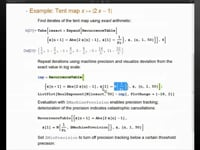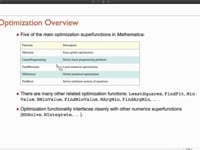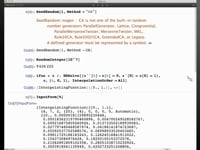Learn how »

# Mathematical Numerics and Special Functions

Oleksandr Pavlyk highlights the advantages of using Mathematica for numeric modeling and computation in this presentation from Mathematica Experts Live: Numeric Modeling in Mathematica.